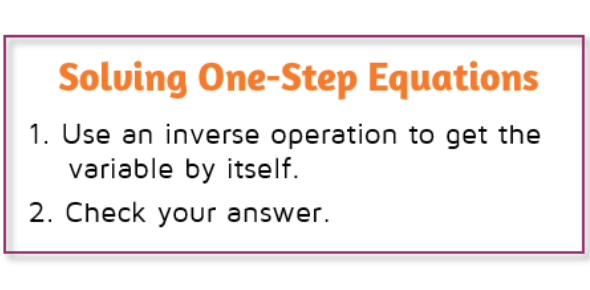# Solving One-step Equations Quiz!

23 Questions | Attempts: 15186
ShareSettingsIt's a simple yet quite practical quiz on one-step equations. For a formal introductory definition on the topic, it's an algebraic equation which is solved in just one step, that's why it's called so. Now, here you are going to face more than 20 questions to shake your brain cells (probably). So, let's get started.

• 1.
What is the inverse operation for multiplication?
• A.

• B.

Subtraction

• C.

Division

• 2.
What is the inverse operation for addition?
• A.

Subtraction

• B.

Multiplication

• C.

Division

• 3.
-15 = x / 4
• A.

-60

• B.

60

• C.

-11

• D.

-19

• 4.
21 + m = 4
• A.

25

• B.

17

• C.

-17

• D.

84

• 5.
6n = 30
• A.

5

• B.

-5

• C.

24

• D.

180

• 6.
9 = x / 18
• A.

-162

• B.

2

• C.

162

• D.

11

• 7.
-5 = -20 + x
• A.

-15

• B.

-25

• C.

100

• D.

15

• 8.
11x = -286
• A.

297

• B.

26

• C.

-297

• D.

-26

• 9.
-5 = x / 12
• A.

50

• B.

-50

• C.

-60

• D.

-17

• 10.
X + 8 = 15
• A.

X = 7

• B.

X = -7

• C.

X = 23

• D.

X = -23

• 11.
Y - 3 = -5
• A.

Y = 8

• B.

Y = -8

• C.

Y = 2

• D.

Y = -2

• 12.
B + 24 = 19
• A.

B = 5

• B.

B = -43

• C.

B = 43

• D.

B = -5

• 13.
3x = 12
• A.

X = -4

• B.

X = 4

• C.

X = 36

• D.

X = 15

• 14.
-2x = 88
• A.

X = 44

• B.

X = -44

• C.

X = -176

• D.

X = 176

• 15.
M + 43 = 100
• A.

M = 57

• B.

M = 143

• C.

M = -143

• D.

M = -57

• 16.
H - 34 = 17
• A.

H = 17

• B.

H = 51

• C.

H = -17

• D.

H = -51

• 17.
7d = 56
• A.

D = -8

• B.

D = 7

• C.

D = 8

• D.

D = 56

• 18.
R / 5 = -9
• A.

R = 5

• B.

R = 9

• C.

R = -45

• D.

R = 45

• 19.
Y / 12 = -11
• A.

Y = -132

• B.

Y = 12

• C.

Y = 1

• D.

Y = 132

• 20.
-3m = -66
• A.

198

• B.

-22

• C.

22

• D.

69

• 21.
9 + x = 2
• A.

7

• B.

-11

• C.

-7

• D.

11

• 22.
-12 = x / 3
• A.

4

• B.

-36

• C.

-4

• D.

36

• 23.
-3 + x = -12
• A.

-15

• B.

15

• C.

-9

• D.

9

## Related TopicsBack to top
×

Wait!
Here's an interesting quiz for you.